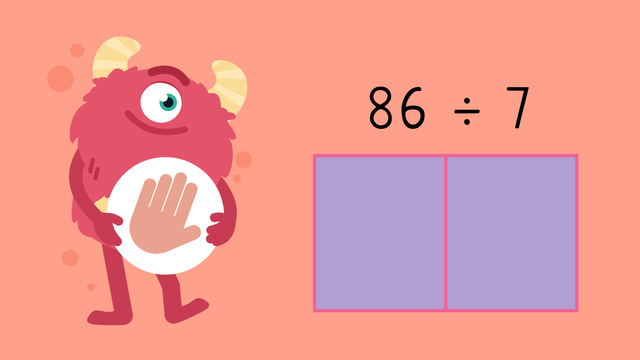# Division with Remainders (Area Models) — Let's Practice!Rating

Ø 5.0 / 1 ratings
The authorsTeam Digital
Division with Remainders (Area Models) — Let's Practice!
CCSS.MATH.CONTENT.4.NBT.B.6

## Basics on the topicDivision with Remainders (Area Models) — Let's Practice!

Today we are practicing division with remainders using area models with Razzi! This video contains examples to help you further practice and grow confident in this topic.

### TranscriptDivision with Remainders (Area Models) — Let's Practice!

Razzi says get these items ready, because today we're going to practice division with remainders, area models. It's time to begin! Use an area model to solve eighty-six divided by seven. Pause the video to work on the problem, and press play when you are ready to see the solution! Seven times ten equals seventy. Eighty-six minus seventy is sixteen. Carry the sixteen over. Seven times two equals fourteen. Sixteen minus fourteen is two, which is the remainder. Ten plus two equals twelve. Add the remainder, two. Did you also get twelve remainder two? Let's tackle one more problem! Use an area model to solve five hundred twenty-seven divided by four. Pause the video to work on the problem, and press play when you are ready to see the solution! Four times one hundred equals four hundred. Five hundred twenty-seven minus four hundred is one hundred twenty-seven. Carry the one hundred twenty-seven over. Four times thirty equals one hundred twenty. One hundred twenty-seven minus one hundred twenty is seven. Carry the seven over. Four times one equals four. Seven minus four is three, which is the remainder. One hundred plus thirty plus one is one hundred thirty-one. Add the remainder, three. Did you also get one hundred thirty-one remainder three? Razzi had so much fun practicing with you today! See you next time!